Click to Chat

1800-1023-196

+91-120-4616500

CART 0

• 0

MY CART (5)

Use Coupon: CART20 and get 20% off on all online Study Material

ITEM
DETAILS
MRP
DISCOUNT
FINAL PRICE
Total Price: Rs.

There are no items in this cart.
Continue Shopping• Complete JEE Main/Advanced Course and Test Series
• OFFERED PRICE: Rs. 15,900
• View Details

Revision Notes on Chemical Thermodynamics:

Basic Terminology:

Terms

Explanation

System

Part of the universe under investigation.

Open System

A system which can exchange both energy and matter with its surroundings.

Closed System

A system which permits passage of energy but not mass, across its boundary.

Isolated system

A system which can neither exchange energy nor matter with its surrounding.

Surroundings

Part of the universe other than system, which can interact with it.

Boundary

Anything which separates system from surrounding.

State variables

The variables which are required to be defined in order to define state of any system i.e. pressure, volume, mass, temperature, surface area, etc.

State Functions

Property of system which depend only on the state of the system and not on the path.

Example: Pressure, volume, temperature, internal energy, enthalpy, entropy etc.

Intensive properties

Properties of a system which do not depend on mass of the system i.e. temperature, pressure, density, concentration,

Extensive properties

Properties of a system which depend on mass of the system i.e. volume, energy, enthalpy, entropy etc.

Process

Path along which state of a system changes.

Isothermal process

Process which takes place at constant temperature

Isobaric process

Process which takes place at constant pressure

Isochoric process

Process which takes place at constant volume.

Process during which transfer of heat cannot take place between system and surrounding.

Cyclic process

Process in which system comes back to its initial state after undergoing series of changes.

Reversible process

Process during which the system always departs infinitesimally from the state of equilibrium i.e. its direction can be reversed at any moment.

Irriversible Process

This type of process is fast and gets completed in a single step. This process cannot be reversed. All the natural processes are of this type

Heat, energy and work:

Heat (Q):

Energy is exchanged between system and surround in the form of heat when they are at different temperatures.

Heat added to a system is given by a positive sign, whereas heat extracted from a  system is given negative sign.

It is an extensive property.

It is not a state function.

Energy:

It is the capacity for doing work.

Energy is an extensive property.

Unit : Joule.

Work (W):

Work = Force × Displacement i.e. dW = Fdx

Work done on the system is given by positive sigh while work done by the system is given negative sign.

Mechanical Work or Pressure-Volume Work: work associated with change in volume of a system against an external pressure.

Work done in reversible process: W=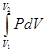W = – 2.303 nRT log v2/v1  =  –2.303 nRT log p1/p2

Wok done in isothermal reversible contraction of an ideal gas:
?W = – 2.303 nRT log v2/v1  =  –2.303 nRT log p1/p2
Unit : Joule.

Internal Energy (E or U):

Sum of all the possible types of energy present in the system.

ΔE  = heat change for a reaction taking place at constant temperature and volume.

ΔE is a state function.

It is an extensive property.

Value of ΔE is -ve for exothermic reactions while it is +ve for endothermic reactions.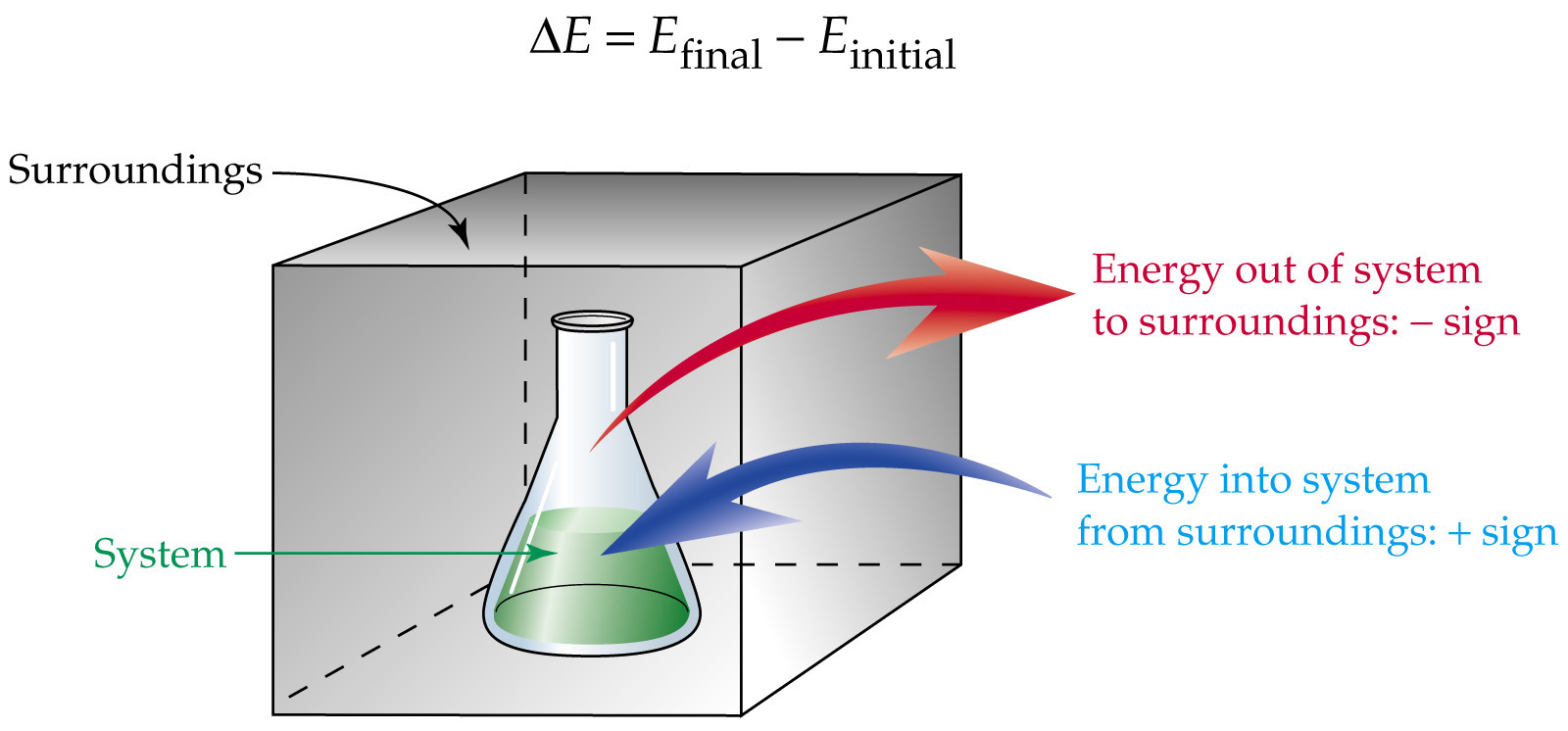First Law of Thermodynamics:

Energy can neither be created nor destroyed although it can be converted from one form to another.

or

Energy of an isolated system is constant.

Mathematical Expression

Heat observed by the system = its internal energy + work done by the system.
i.e. q = dE + w

For an infinitesimal process

dq = dE + dw

Where, q is the heat supplied to the system and w is the work done on the system.

For an ideal gas undergoing isothermal change ΔE =0.

so q= -w.

For an isolated system, dq=0

so, dE = - dw

For system involving mechanical work only

ΔE = q - pdV

At constant volume i.e. isochoric process

ΔE = qv

For Isothermal Process

ΔE = 0

or

q = - pdV  =-W

?q = 0

or

ΔE = W

Enthalpy (H):

H = E+PV

At constant pressure:

dH = dE + pdV

For system involving mechanical work only

dH = QP (At constant pressure)

For exothermic reactions:

dH = -ve

For endothermic reactions:

dH = +ve

Relation between dH and dE:

dH = dE + dng RT

Where,

dng = (Number of moles of gaseous products - Number of moles of gaseous reactants)

Heat capacity:

Amount of heat required to rise temperature of the system by one degree.

C = q / dT

Specific heat capacity: Heat required to raise the temperature of 1 g of a substance by one dgree.

Cs = Heat capacity / Mass in grams

Molar heat capacity: Heat required to raise the temperature of 1 g of a substance by one dgree.

Cm = Heat capacity / Molar mass.

Heat capacity of system at constant volume:

Cv  =  (dE/dT)v

Heat capacity of system at constant pressure:

Cp  =  (dE/dT)p

Cp – Cv = R

Variation Of Heat Of Reaction With Temperature:

dCP = (dH2 - dH1)/(T2-T1)   &    dCV = (dE2 - dE1)/(T2-T1

Bomb Calorimeter:

?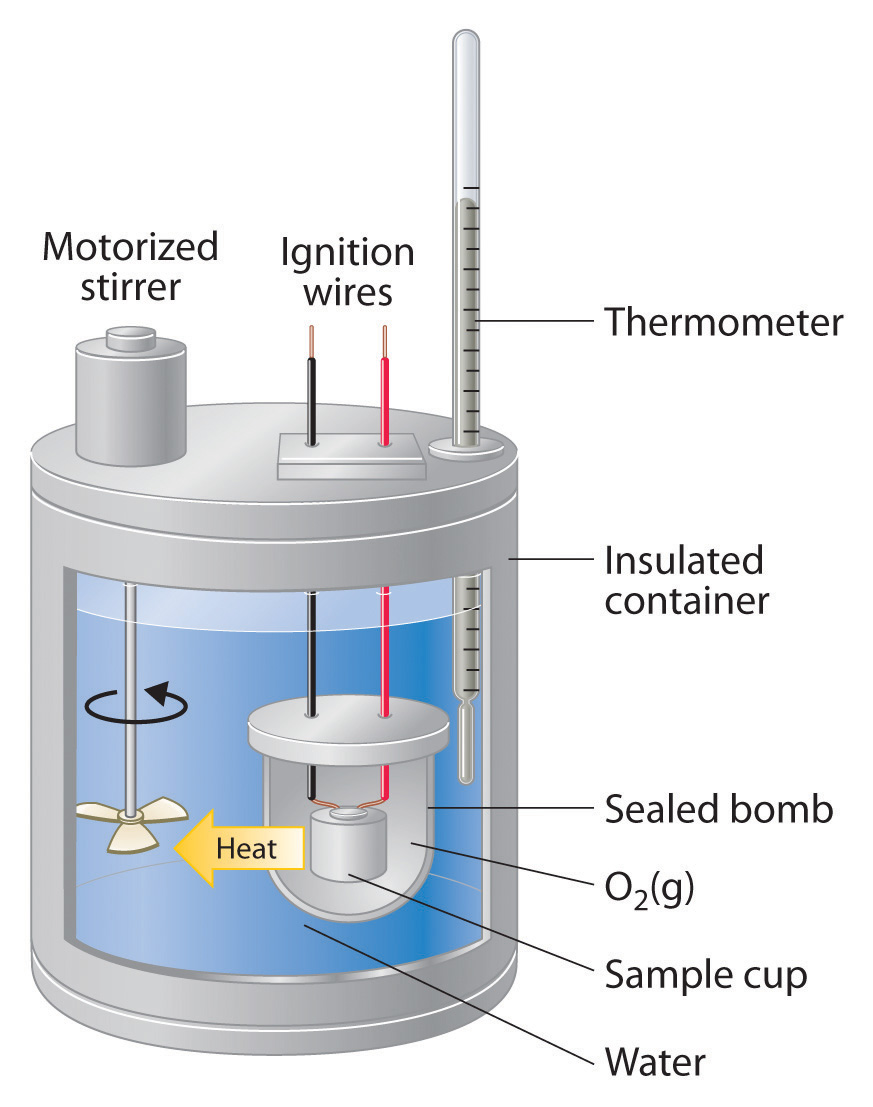Heat exchange = Z × ΔT

Z–Heat capacity of calorimeter system

ΔT– Rise in temp.

Heat changes at constant volumes are expressed in ΔE and Heat changes at constant

pressure are expressed in dH.

Enthalpies of Reactions:

Enthalpies

Definitions

Example

Enthalpy of Formation

Enthalpy change when one mole of a given compound is formed from its elements

H2(g) + 1/2O2(g) →  2H2O(l),
ΔfH = –890.36 kJ / mol

Enthalpy of Combustion

Enthalpy change when one mole of a substance is burnt in oxygen.

CH4 + 2O2(g) →CO2  + 2H2O(l),
ΔcombH  = –890.36 kJ / mol

Enthalpy of Neutralization

Enthalpy change when one equivalent of an acid is neutralized by a base in dilute solution.

H+ (aq) + OH– (aq) → H2O(l)
ΔneutH = –13.7 kcal

Enthalpy of Hydration

Enthalpy change when a salt combines with the required number of moles of water to form specific  hydrate.

CuSO4(s) + 5H2O (l) → CuSO45H2O,

ΔhydH° = –18.69 kcal

Enthalpy of Transition

Enthalpy change when one mole of a substance is transformed from one allotropic form to another allotropic form.

C (graphite) → C(diamond),                      ΔtransH° = 1.9 kJ/mol

Enthalpy of Sublimation

Enthalpy change when one mole of a solid substance sublime at constant temp. and 1 bar pressure

CO2(S) → CO2(g)
ΔtfusH° = 6.00 kJ/mol

Enthalpy of fusion

Enthalpy change when one mole of a solid melts

H2O(S) → H2O (l)
ΔtsubH° = 73.00 kJ/mol

Hess’s Law of constant heat summation:

?The total enthalpy change of a reaction is the same, regardless of whether the reaction is completed in one step or in several steps.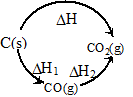According to Hess’s law: ΔH = ΔH1 + ΔH2

Born–Haber Cycle: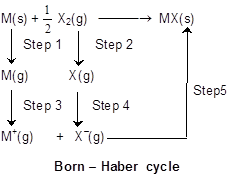Applying Hess’s law we get

ΔH1 + 1/2 ΔH2 + ΔH3 + ΔH4 + ΔH5 = ΔHf (MX)  (Lattice energy)

Lattice energy: The change in enthalpy that occurs when 1 mole of a solid crystalline substance is formed from its gaseous ions.

Second law of thermodynamics

Statement:

It is impossible to take heat from a hot reservoir and convert it completely into work by a cyclic process without transferring a part of it to a cold reservoirs.

Mathematically:

ΔS =  qrev/T

Where,

ΔS is entropy change.

Entropy is the degree of randomness thus it increases with increase in randomness of particles of the system i.e.  ΔS is positive for melting of ice.

At equilibrium, ΔS = 0

For a spontaneous process, ΔS > 0

Entropy change in an isothermal reversible expansion of a gas

Spontaneous Processes: These type of physical and chemical changes occur of its own under specific circumstances or on proper initiations. For example: Flow of liquids from higher to lower level.

Gibbs free energy(ΔG):

ΔG = ΔH - TΔS

ΔG = nRT ln Keq

ΔG = nFEcell

At equilibrium, ΔG = 0

For spontaneous process, ΔG < 0

Bond Energies:

Average amount of energy required to break one mole bonds of that type in gaseous molecules.

H–OH(g) → 2H(g) + ½O(g)                ΔH = 498 kJ

O–H(g) → H2(g) + ½O2 (g)         ΔH = 430 Kj

ΔHO–H = (498 + 430)/2 = 464 kJ mol–1

Efficiency of a heat engine (carnot cycle):

W = R (T2 – T1) ln v2/v1

q2 = RT2 ln v2/v1

W = q2Efficiency (h). h =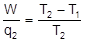### Course Features

• 728 Video Lectures
• Revision Notes
• Previous Year Papers
• Mind Map
• Study Planner
• NCERT Solutions
• Discussion Forum
• Test paper with Video Solution